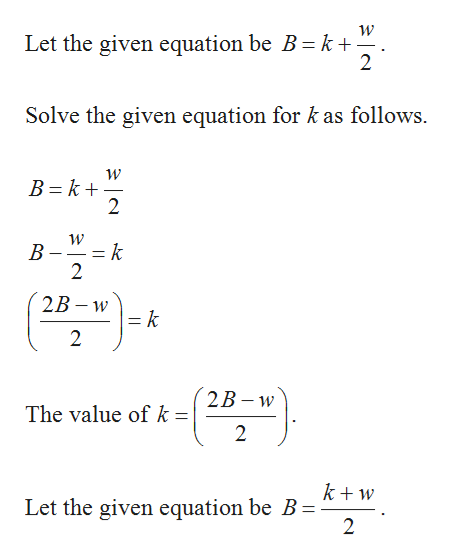# B= k+w/2 solve for K

Question

B= k+w/2

solve for K

check_circleExpert Solution
Step 1help_outlineImage TranscriptioncloseW Let the given equation be B = k+' 2 Solve the given equation for k as follows. B k 2 W k В 2 2B w k 2 2В - w The value of k: 2 k+w Let the given equation be B = 2 fullscreen

### Want to see the full answer?

See Solution

#### Want to see this answer and more?

Solutions are written by subject experts who are available 24/7. Questions are typically answered within 1 hour*

See Solution
*Response times may vary by subject and question
Tagged in

### Other